{{announcement.body}}
{{announcement.title}}

# Performance Evaluation of Java ArrayLists

DZone 's Guide to

# Performance Evaluation of Java ArrayLists

### Let's do a performance breakdown of the ArrayList add operation to see how to get the most throughout out of your ArrayLists.

· Performance Zone ·
Free Resource

Comment (0)

Save
{{ articles.views | formatCount}} Views

In this article, we will look at the performance characteristics of the Java ArrayList (java.util.ArrayList) add operation.

An ArrayList has an initial capacity, which is simply the size of the array used to store the elements in the list. When you create an ArrayList you can specify the initial capacity. For example:

``ArrayList<Integer> arrayList = new ArrayList<>(100);``

In this case, the initial size of the ArrayList will be 100. As you add elements to an ArrayList, its capacity grows automatically. The growing factor is 1.5. If we do not specify an initial capacity then an ArrayList object will be created containing an initial array of size ten.

``ArrayList<Integer> arrayList = new ArrayList<>();``

## Performance Evaluation

In this section, we will provide details of how we conducted our experiments. The performance tests are done using JMH (Java Microbenchmark Hardness). It is a tool that can be used to implement benchmarks correctly for the applications run top of the JVM.  JVM's HotSpot VM has the ability to do optimizations like dead code elimination.

The two main performance metrics considered are

1. Average latency (measured in nanoseconds)

2. Throughput (measured in number of operations per millisecond)

The scenario implemented is sequentially adding elements to an ArrayList using the add operation. We run the benchmark by varying the following parameters:

1. Initial capacity (size) of the ArrayList

2. Final size of the ArrayList (the maximum number of elements used)

The part of the benchmark code is shown below:

``````…………….

@Benchmark

ArrayList < Integer > m;

m = new ArrayList < > (initialCapacity);

for (int j = 0; j < finalSize; j++) {

}

bh.consume(m);

count += 1;

}

………….``````

The following are the parameters specified for JMH, which are fixed for all runs.

1. Number of warmup iterations: 10

2. Numbers of iterations: 10

3. Number of forks: 2

5. Time unit ns (nanoseconds)

6. Mode: benchmark mode

The performance test was run on a 4-core machine with 8 GB RAM and JDK used was Oracle 1.8_131

## Performance Results

This section presents the performance results. These are the results obtained:

 Final size Initial Size Average Latency (ns) Throughput (operations/ms) 9 10 79.53 13045.111 100 92.945 10471.726 1000 345.138 2957.518 10000 2066.279 495.057 99 10 675.109 1506.031 100 440.578 2244.508 1000 669.46 1378.31 10000 2542.431 426.257 999 10 5797.814 171.068 100 5583.3 170.748 1000 4145.378 232.636 10000 5995.691 171.921 9999 10 54751.808 18.212 100 53713.615 17.492 1000 50556.323 19.876 10000 41174.069 24.14

Let's now make a few plots so that we can understand the behavior. The following graph shows how the average latency varies with the initial array size when the final size is 99.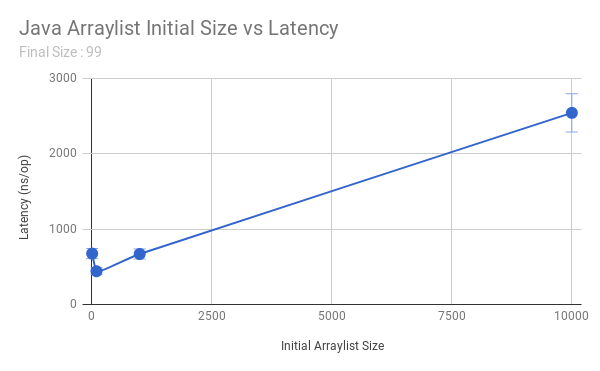The following graph shows how the throughput varies with the initial array size when the final size is 99.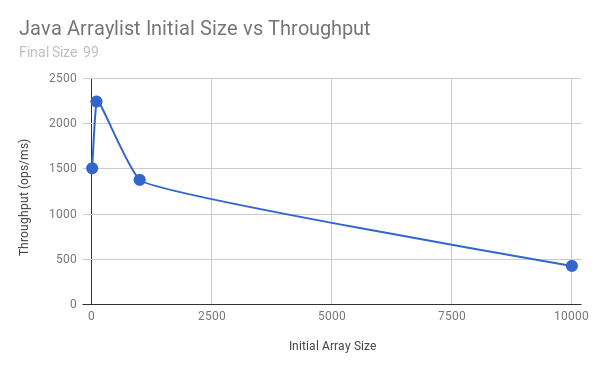The following graph shows how the average latency varies with the initial array size when the final size is 999.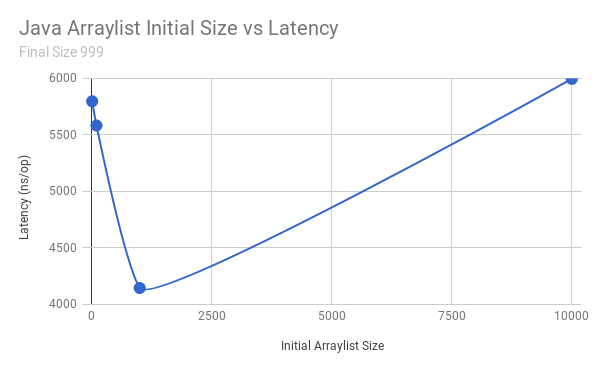The following graph shows how the throughput varies with the initial array size when the final size is 999.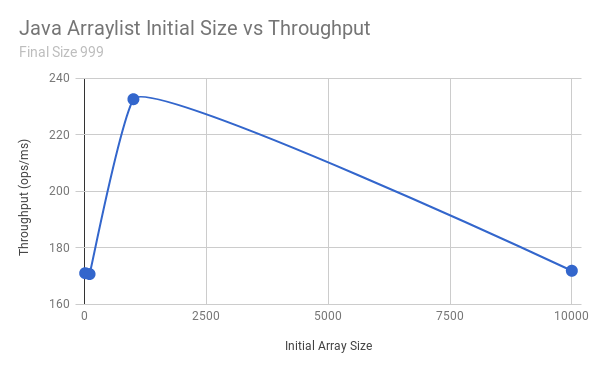The following graph shows how the average latency varies with the initial array size when the final size is 9999.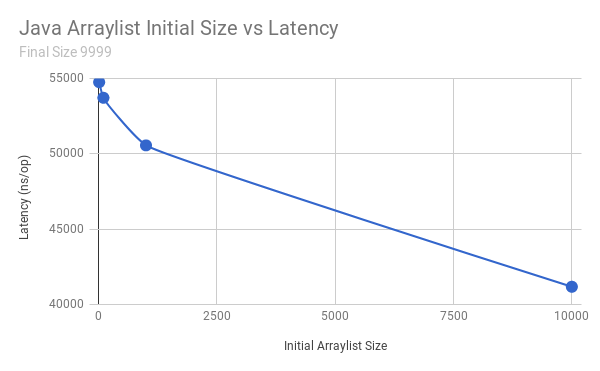The following graph shows how the throughput varies with the initial array size when the used capacity is 9999.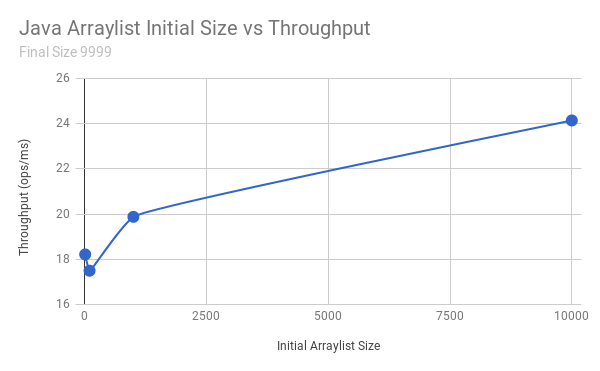## Discussion

### Initial array size > Final size

If the initial array size is greater than the final size, there can be a significant degradation in performance.

For example, when we create an array with an initial capacity of 1000 and use only the first 10 elements, we observed an average latency of 345 ns. On the other hand, when we create an array with an initial capacity of 10 and used the first 10 elements, then we get an average latency of 57 ns.

The main reason for the degradation in performance for the larger initial array size case is the cost of initializing the large array itself. When we profile applications, we noticed array initialization taking a significant amount of processing time.

### Initial Array Size < Final Size

If the initial size is smaller than the final size, then there can also be a significant degradation in performance.

For example, when we create an array with an initial capacity of 10 and use the first 1000 elements, then we observed an average latency of 5797 ns. On the other hand, when we create an array with an initial capacity of 1000 and use 1000 elements, then we get an average latency of 4145 ns.

The main reason behind the degradation in performance for the smaller initial array size case is the additional processing needed for copying existing elements into a new array with a large size together. When we profile benchmark code, we noticed array copy operation taking a significant amount of processing time. In addition to this, there may be degradation due to the creation of arrays multiple times and initializing them.

### Over Specifying vs. Under Specifying Initial Array Size

We note that the degradation in performance, as a result of over specifying the initial size, is less compared to that of under specifying. Let's now discuss this behavior in a bit more detail. Let's assume that the final size of the array list is 1000. The following figure plots the variation in the throughput with increasing ArrayList size.We note that the maximum throughput is achieved when the initial array list size is 1000 (i.e. when initial size = final size). Let us now assume that TPS_1 and TPS_2 represent the throughput if the initial array size is 500 and 1500 respectively (refer to the figure above). We note that TPS_2 is significantly higher than TPS_1, which validates our claim regarding the performance differences in over specifying vs. under specifying the initial size.

## Conclusion

It is clear from the from the results (considering the add operation of ArrayList) that if the required maximum capacity of the ArrayList is known, we can get the optimal performance (both average latency and throughput) by specifying the initial capacity to the required capacity.

In doing so, we can get 24% to 34% improvement in average latency and 30% to 50% improvement in throughput. In our future work, we hope to consider benchmarking other operations such as insert, remove, and get in addition to the add operation. In our current tests, although we vary the final size between different tests, for a given test scenario final size is fixed. We hope to extend our work to cater for scenarios where the final size varies. In such cases, the final size can come from probability distribution, which represents (actual) access pattern of users.

Topics:
java ,performance analysis ,java performance ,arraylist

Comment (0)

Save
{{ articles.views | formatCount}} Views

Opinions expressed by DZone contributors are their own.